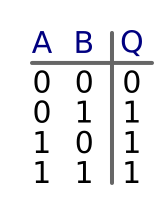# OR gates

Martin McBride, 2017-01-04
Tags or gate truth table
Categories logic logic gates

An OR gate has two inputs and one output. It is similar to an AND gate, except that the output obeys different rules.

The two inputs can each have values 0 or 1.

The state of the inputs controls the state of the output. The output value is 1 if either (or both) inputs are 1, otherwise it is 0. It is called an OR gate because the output will be 1 if input A OR input B is one.

## Symbol

Here is the symbol for an OR gate. The inputs are labelled A and B, the output is labelled Q.## Truth table

A truth table is a table which shows all possible combinations of inputs (A and B), and the resulting value of the output, Q. Here is the truth table for an OR gate, as you can see the output is 1 if either (or both) inputs are 1.